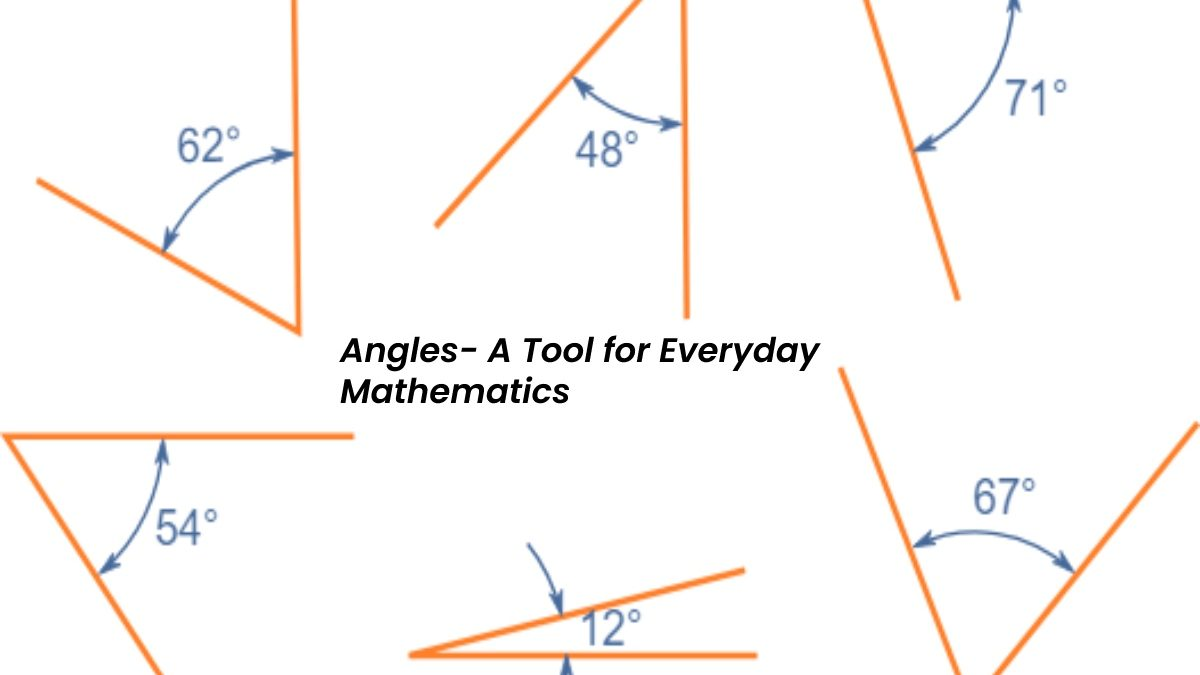28 Sep 2022

## Blog Post# Angles- A Tool for Everyday Mathematics

Angles – Mathematics has played a huge role in revolutionizing our world. We can find applications of math in our daily life. Some great mathematicians have brought great solutions to the rarest of problems and have left their mark in the field of mathematics. Most people think that in math we have to deal only with numbers, but it’s much more than that, it is a subject that deals with patterns, numbers and shapes. It is also considered one of the toughest subjects in the world by many students.  In math, people have to deal with complex calculations. From the start of academics, every student has to study math because of its applications in the real world. Maths is used everywhere from counting simple figures to rocket science, science plays a huge role in every field. The main branches that math deals with are algebra, number theory, statistics, calculus, geometry and arithmetic. From the above-mentioned branches, we are going to discuss a few important topics of geometry in detail. Geometry in general deals with shapes, sizes and angles. One such topic in geometry is the angle. Angles can be divided into various categories such as acute angle, obtuse angle, right angle, supplementary angles, complementary angles and many more.

In most cases, an angle is measured in degrees or radians. Most of the shapes have some constant angle like in an equilateral triangle all three angles are 60 degrees, in a rectangle and square all sides are fixed at an angle of 90 degrees with each other. Two pairs of lines are called parallel if the angle between them is zero degrees and are said to be perpendicular to each other when the angle between the two lines is ninety degrees. There is a lot of classification of [angles] as discussed above, we are going to discuss complementary angles in detail.

## Complementary Angle:

When the total of two angles equals ninety degrees, the pair of angles is said to be complementary, or one can even define complementary angles as a pair of [angles] whose sum is equal to ninety degrees. One should always keep in mind that in some conditions even though the sum of angles is equal to 90 degrees, but they are a sum of more than two angles then they are not considered as complementary angles. Let us take an example, let there be a 20-degree angle and a 70-degree angle. We can see that the sum of the supplied angles is 90 degrees, indicating that they are complementary [angles], with 20 degrees being the complement of 70 degrees and vice versa. There are two types of [complementary angles]. Adjacent and nonadjacent. Let’s talk about both of them.

• Adjacent complementary [angles]: They are defined as two complementary angles that share a common vertex and a common line. If two [angles] are not considered as non-adjacent [complementary angles] then they have to be adjacent complementary [angles].
• Non-adjacent complementary [angles]: If two given angles don’t have any common point and common line, they are said to be non-adjacent [complementary angles]. One can also say that if two [angles] add up to 90 degrees and aren’t adjacent complementary angles, then they’re surely considered non-adjacent [complementary angles].

In the above article, we have tried to discuss all the concepts related to corresponding angles. Nowadays, with an increase in the online mode of study, people can find several platforms to gather information. One such platform is Cuemath. It is one of the finest platforms to make our math-related problems crystal clear. Its language is easily understandable. One will get thousands of topics related to math to read on it. Not only school or college students, but an individual of any age can make the best use of this platform by gaining enormous knowledge available on it. Learning from online mode has increased enormously in the last few years. Studying from such online platforms not only saves our energy but also helps us to save time. One should take complete benefit of such platforms.